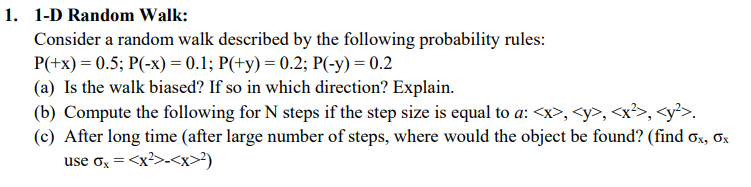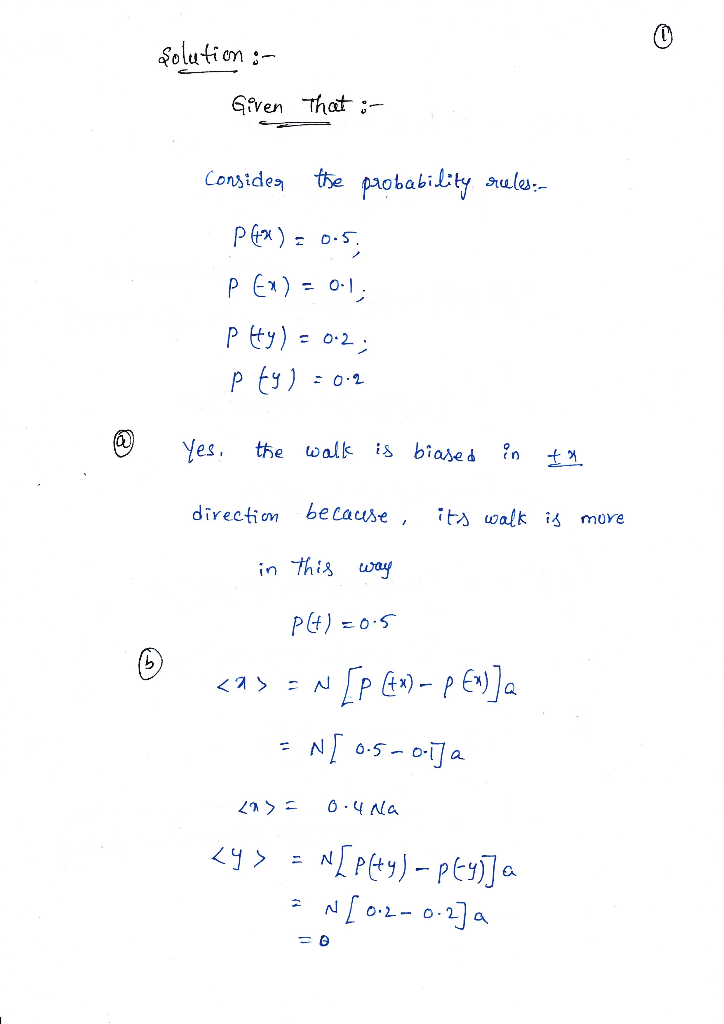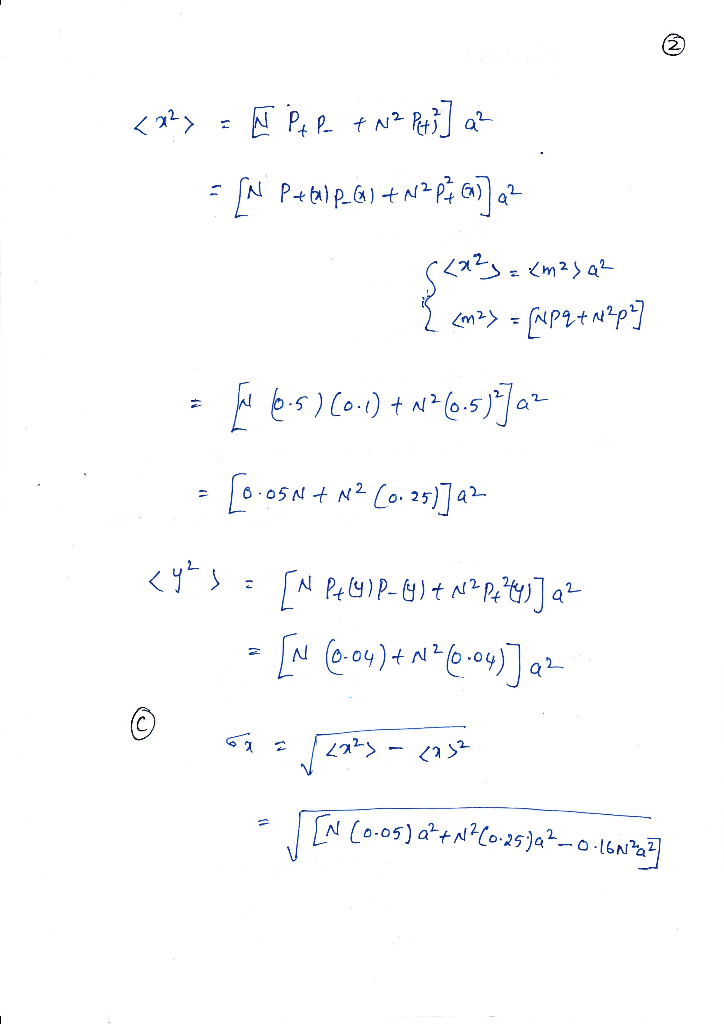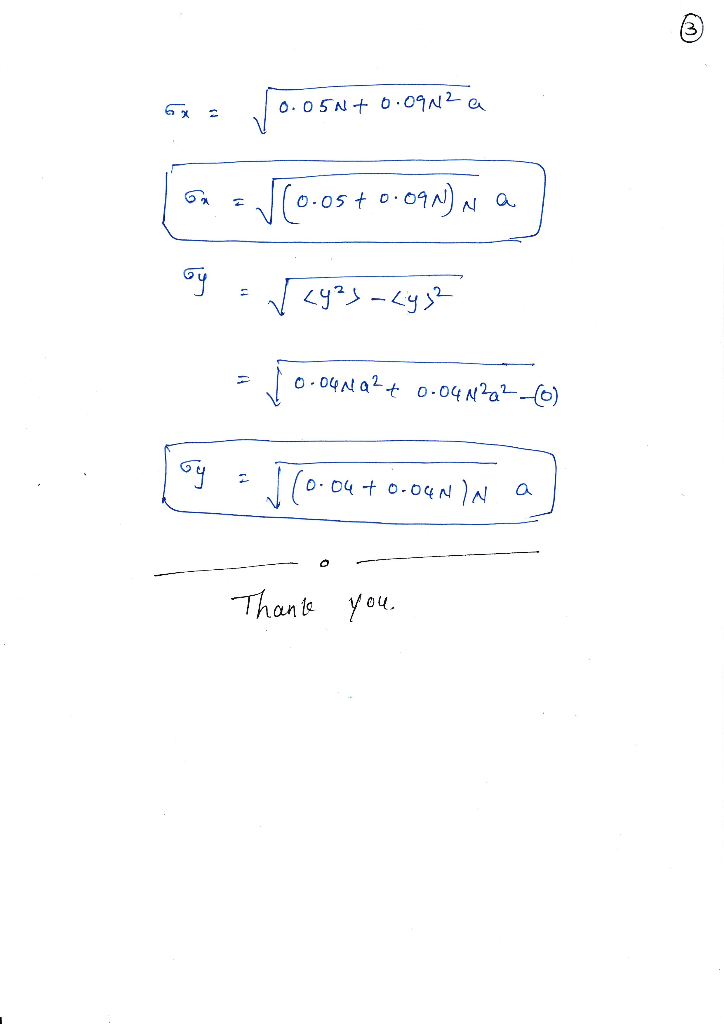# 1-D Random Walk: Consider a random walk described by the following probability rules: P(+x) = 0.5...1-D Random Walk: Consider a random walk described by the following probability rules: P(+x) = 0.5; P(-x) = 0.1 ; P(ty) = 0.2; P(-y) = 0.2 (a) Is the walk biased? If so in which direction? Explain. (b) Compute the following for N steps if the step size is equal to a: , , (c) After long time (after large number of steps, where would the object be found? (find Ox, Ox I.

Solution:##### Add Answer of: 1-D Random Walk: Consider a random walk described by the following probability rules: P(+x) = 0.5...
More Homework Help Questions Additional questions in this topic.